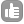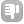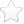0Let me consider a proton experiment done on a 500 MHz spectrometer. The signal coming from the sample has a frequency of approximately 500 MHz. Then the mixer converts the signal down to a signal in the kHz region by subtracing the reference frequency. This seems clear to me. Now, what appens in the second dimension? Let me take a COSY spectrum as an example. Let me call t1 the evolution time in the second dimension. Which is the frequency of the signal in this dimension? Is it in the kHz or in the MHz range? Every book I look at writes the evolution in the second dimension as if it was in the kHz region (proton frequency minus reference frequency), but I don't understand how is this possible because i don't see any machinery that suctracts the reference from the signal in the second dimension. Thaks in advance asked Nov 12 '14 at 08:03PQdotL 21 The frequencies in "the other dimension" are built up point by point by varying the t1 delay after every experiment. So both sets of frequency information are present in the FID and are presented referenced to the carrier frequency. - dstephenson (Dec 16 '14 at 06:27)

Be the first one to answer this question!